### IMO Shortlist 2011 problem N4

Kvaliteta:
Avg: 0,0
Težina:
Avg: 7,0
For each positive integer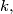$k,$ let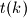$t(k)$ be the largest odd divisor of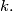$k.$ Determine all positive integers$a$ for which there exists a positive integer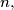$n,$ such that all the differences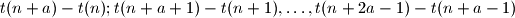are divisible by 4.

Proposed by Gerhard Wöginger, Austria
Izvor: Međunarodna matematička olimpijada, shortlist 2011